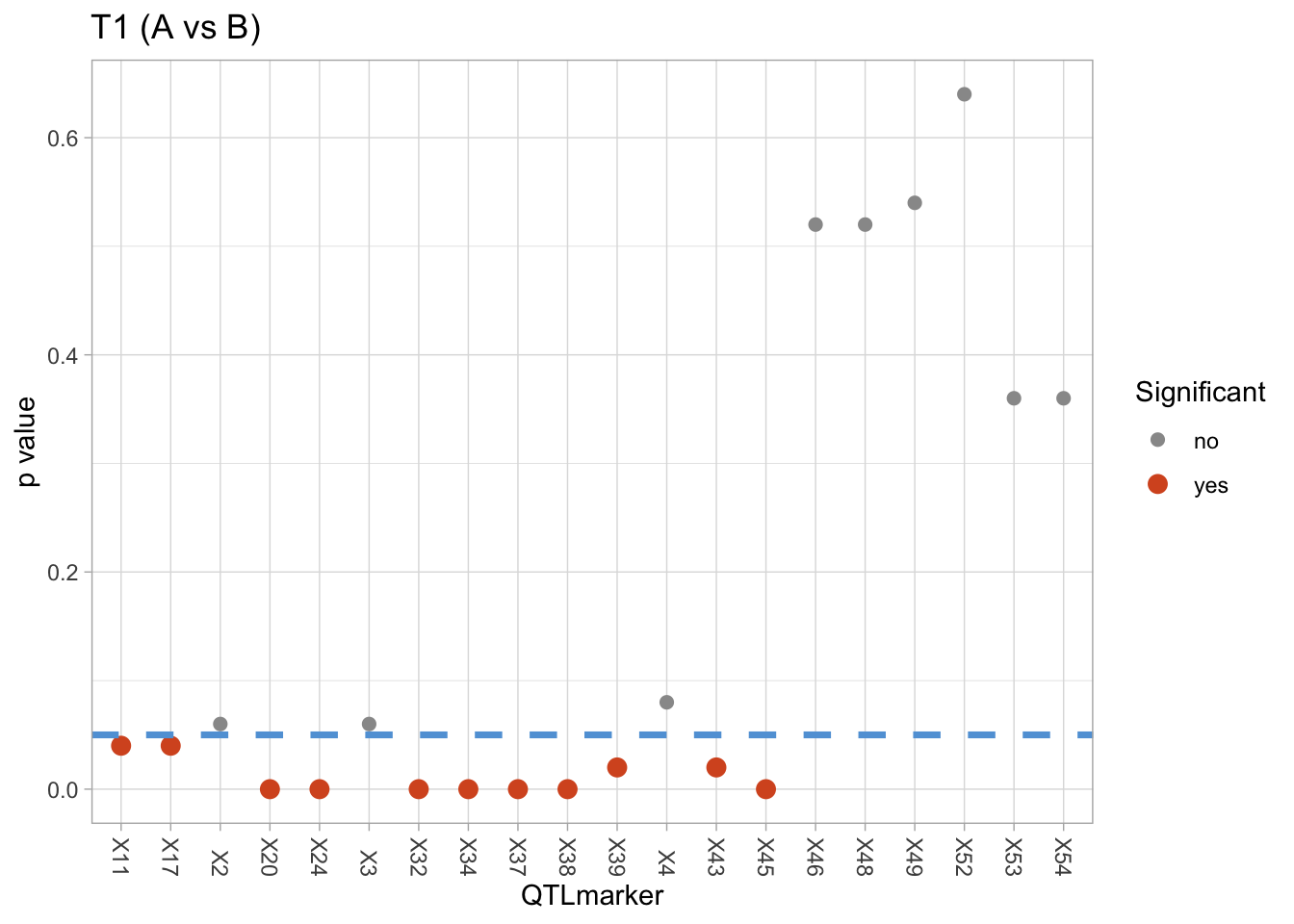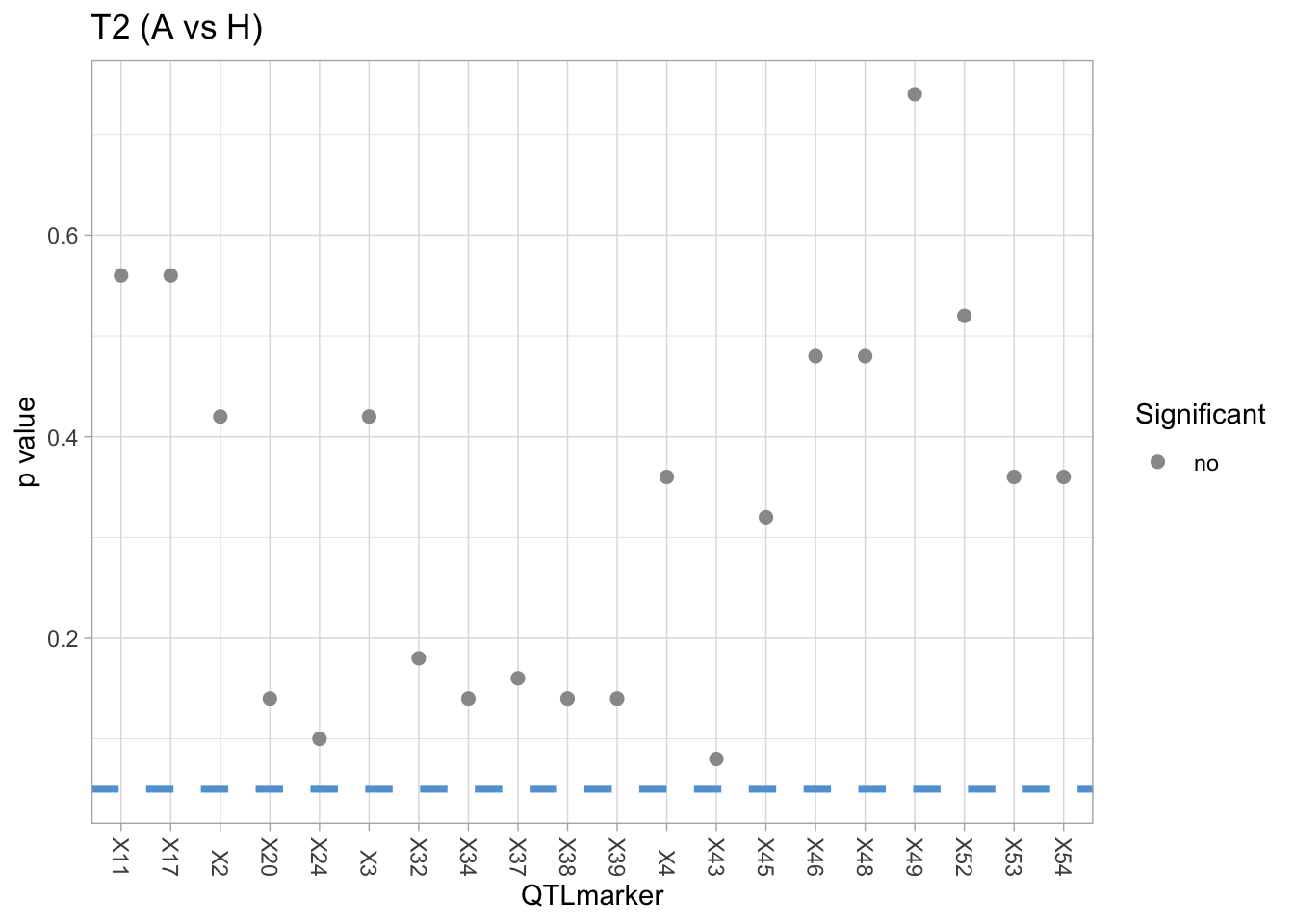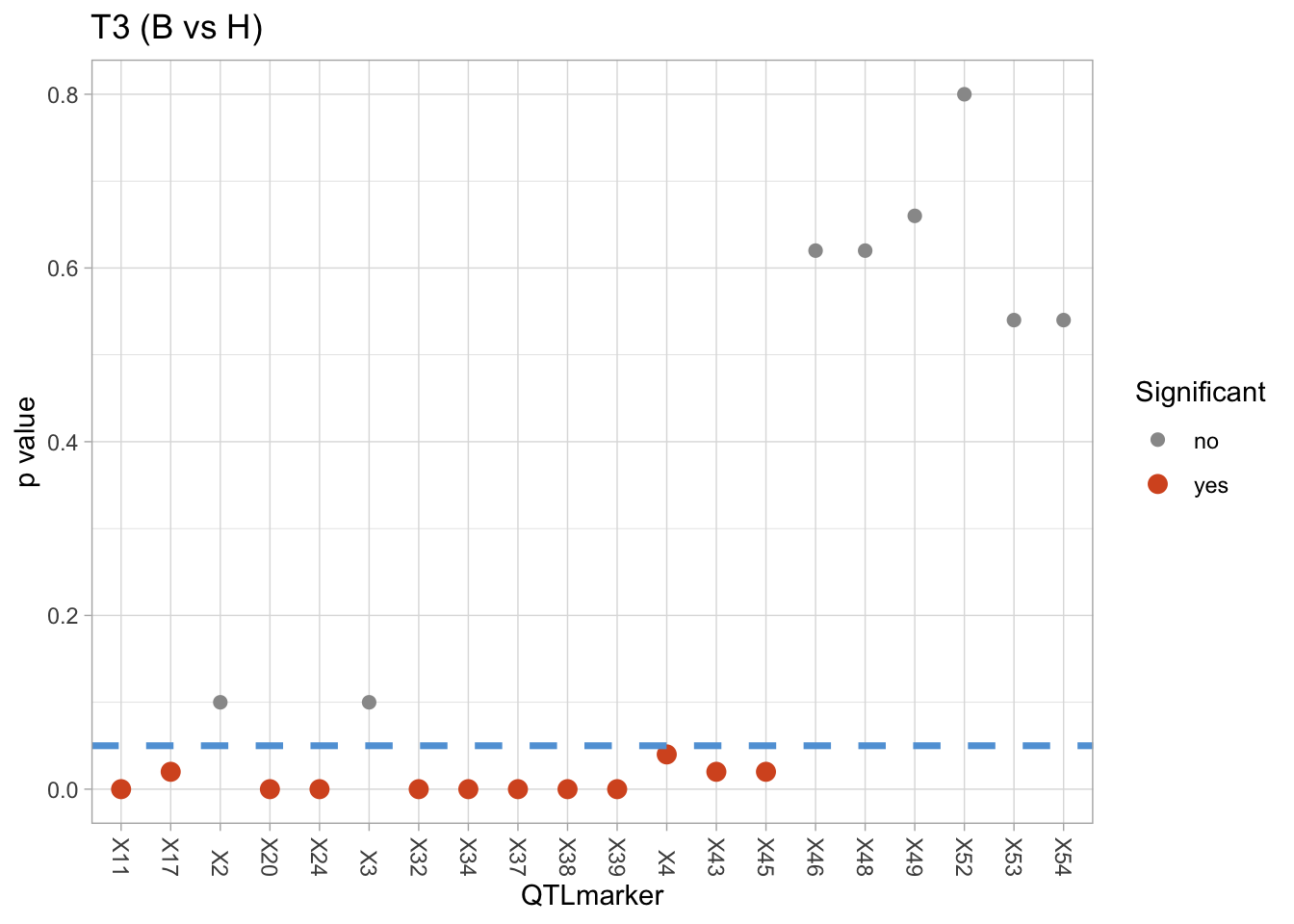## 1. Introduction

This file implement a preliminary network analysis on the expression data in “MergedTagseqQTL.csv”. The goal of the analysis is to identify the QTLmarkers which have significant effects to the expression networks.

Specifically, the dataset include the expression data on $$p = 25845$$ genes of $$n = 351$$ observations with $$m = 234$$ QTLmarkers. For the $$i$$-th QTLmarker where $$i = 1,...,m$$, there are three possible genotypes “A”,“B” , and “H” with corresponding expression networks $$N_{iA}, N_{iB}, N_{iH} \in \mathbb{R}^{p \times p}$$. We detect whether there are significant differences among these three networks $$N_{iA}, N_{iB}, N_{iH}$$.

We apply the sparse-Leading-Eigenvalue-Driven (sLED) test in Zhu et al 2017 for the following hypothesis tests: $(T_1) \quad H_0: N_{iA} = N_{iB} \quad \leftrightarrow \quad H_1: N_{iA} \neq N_{iB}$ $(T_2) \quad H_0: N_{iA} = N_{iH} \quad \leftrightarrow \quad H_1: N_{iA} \neq N_{iH}$ $(T_3) \quad H_0: N_{iB} = N_{iH} \quad \leftrightarrow \quad H_1: N_{iB} \neq N_{iH}$ for all $$i = 1,...,m$$.

Note that this file aims to illustrate the practical usage of sLED test and provide a possible pipeline of gene expression network analysis. The results obtained by the following analyses may not have biological meanings. More preprocessing procedures and external knowledge are necessary for future analysis.

## 2. Setup

The package for sLED is avaliable at https://github.com/lingxuez/sLED.

# load package

library(sLED) #sLED

# sLED installation
# library(devtools)
# devtools::install_github("lingxuez/sLED")

library(ggplot2) # for visualization
library(igraph) # for network visualization

Here, we only consider the subset of the expression data with the gene expressions whose cumulative values $$> 250000$$ due to the computational feasibility of local PC. More sophiscated preprocessing procedures should be applied here.

# load data

qtlmarker <- dat[, 113:346] # genotype of each QTLmarker
tagseq <- dat[,441:(ncol(dat)-2)] # expression data

dim(qtlmarker)
##  351 234
dim(tagseq)
##    351 25845
qtlmarker[1:3, 1:10]
##   X2 X3 X4 X11 X17 X20 X24 X32 X34 X37
## 1 AB AB AB  AB  AB  AB  AB  AB   -   -
## 2 AA AA AA  AA  AA  AA  AA  AA  AA  AA
## 3 AA AA AA  AA  AA  AA  AA  AA  AA  AA
tagseq[1:3, 1:10]
##   ENSGACT00000000002.1 ENSGACT00000000003.1 ENSGACT00000000004.1
## 1                    1                    0                    0
## 2                    3                    0                    0
## 3                    0                    2                    0
##   ENSGACT00000000005.1 ENSGACT00000000006.1 ENSGACT00000000007.1
## 1                    0                    6                    0
## 2                    0                    7                    0
## 3                    0                    2                    0
##   ENSGACT00000000008.1 ENSGACT00000000009.1 ENSGACT00000000010.1
## 1                    1                    0                    0
## 2                    0                    0                    0
## 3                    0                    0                    1
##   ENSGACT00000000011.1
## 1                   26
## 2                   21
## 3                  101
# Trim out rare gene reads
meanreads <- colSums(tagseq, na.rm = T)
# 250000 is just a random number to make the code computationally feasible on local PC
sum(meanreads > 250000)
##  100

## 3. Analysis: Detecting significant QTLmarkers

### Toy example

Before we go to the analysis, we consider a toy example to get familiar with the software and sLED test.

markergeno <- qtlmarker[,1]

# Subdivide expression data by genotype
tagseq_aa <- tagseq[markergeno == "A",]
tagseq_bb <- tagseq[markergeno == "B",]

dim(tagseq_aa)
##   51 100
dim(tagseq_bb)
##   44 100
tagseq_aa[1:3, 1:10]
##    ENSGACT00000000197.1 ENSGACT00000000446.1 ENSGACT00000000615.1
## 43                  351                 1983                 1572
## 48                 1567                 2496                 2351
## 50                 1093                 1826                 2174
##    ENSGACT00000000764.1 ENSGACT00000000955.1 ENSGACT00000001456.1
## 43                  974                 1209                 1044
## 48                 1806                 1877                 1526
## 50                 1922                 1790                 1471
##    ENSGACT00000002285.1 ENSGACT00000002668.1 ENSGACT00000002893.1
## 43                  531                 1778                  836
## 48                  938                 2464                 1633
## 50                 1224                 2050                 1809
##    ENSGACT00000003550.1
## 43                 1737
## 48                 2070
## 50                 1928
tagseq_bb[1:3, 1:10]
##    ENSGACT00000000197.1 ENSGACT00000000446.1 ENSGACT00000000615.1
## 46                  343                 1895                 1438
## 49                 1397                 1335                 2189
## 53                 1161                 2442                 2475
##    ENSGACT00000000764.1 ENSGACT00000000955.1 ENSGACT00000001456.1
## 46                 1102                 1120                 1145
## 49                 1853                 1644                 1707
## 53                 2032                 2052                 1496
##    ENSGACT00000002285.1 ENSGACT00000002668.1 ENSGACT00000002893.1
## 46                  789                 1688                 1275
## 49                 1350                 1899                 1753
## 53                 1287                 2430                 2378
##    ENSGACT00000003550.1
## 46                 1532
## 49                 1755
## 53                 2359
# no need to calculate covariance matrices
sLED_ab = sLED(tagseq_aa, tagseq_bb, sumabs.seq = 0.3, npermute = 20, seeds = 1:20) # T1
## 20 permutation started:
## 10 ,20 ,permutations finished.
sLED_ab$pVal ##  0.2 sLED_ab$leverage
##      [,1] [,2]         [,3] [,4]         [,5] [,6] [,7] [,8]         [,9] [,10]
## [1,]    0    0 6.922978e-06    0 6.832491e-06    0    0    0 2.692252e-06     0
##             [,11] [,12]        [,13]       [,14] [,15]        [,16] [,17] [,18]
## [1,] 3.386729e-05     0 0.0001230776 0.000855468     0 0.0005143463     0     0
##             [,19] [,20]        [,21]        [,22]        [,23] [,24] [,25]
## [1,] 8.868752e-06     0 1.260819e-05 2.717525e-06 0.0001935182     0     0
##      [,26] [,27] [,28]        [,29] [,30] [,31] [,32] [,33] [,34] [,35]
## [1,]     0     0     0 2.977939e-07     0     0     0     0     0     0
##             [,36] [,37] [,38]        [,39] [,40] [,41] [,42] [,43]        [,44]
## [1,] 9.141847e-05     0     0 1.561565e-05     0     0     0     0 0.0006794693
##      [,45] [,46]        [,47]        [,48]        [,49]        [,50]      [,51]
## [1,]     0     0 0.0007713065 3.100449e-05 0.0001450509 0.0006887421 0.01181551
##      [,52] [,53] [,54]       [,55]      [,56]     [,57]     [,58] [,59]
## [1,]     0     0     0 0.005564744 0.06497563 0.2587572 0.4466468     0
##          [,60]        [,61] [,62]        [,63]        [,64] [,65] [,66] [,67]
## [1,] 0.1207375 0.0007818078     0 1.265257e-05 0.0003411658     0     0     0
##      [,68]        [,69]        [,70] [,71]       [,72]        [,73] [,74]
## [1,]     0 1.938333e-05 0.0003259561     0 0.001761228 0.0007786956     0
##             [,75] [,76] [,77]        [,78] [,79]        [,80]        [,81]
## [1,] 0.0004032358     0     0 0.0005818846     0 0.0001431539 0.0006368413
##      [,82]        [,83]        [,84] [,85]        [,86] [,87] [,88]
## [1,]     0 0.0009633652 6.682307e-05     0 0.0003695649     0     0
##             [,89]        [,90]      [,91]      [,92] [,93]        [,94] [,95]
## [1,] 0.0004775586 6.562697e-06 0.06055094 0.00840276     0 0.0001569639     0
##            [,96]       [,97]        [,98]       [,99] [,100]
## [1,] 0.002151067 0.007692146 0.0002414428 0.000453634      0

### Analysis

Here we perform the test for the first $$m_0 = 20$$ QTLmarkers for demonstration.

Sparsity level is the key tuning parameter the sLED test. A smaller sparsity level leads to a smaller number of top genes. Permutation time is another tuning parameter. More permutations lead to a more accurate test result but require more computational resources.

We consider 4 possible sparsity level $$0.1, 0.2, 0.3, 0.5$$ and 50 times of permutations here. The following chuck of codes needs about 15 mins.

s_seq = c(0.1, 0.2, 0.3, 0.5) # sparsity level
m0 = 20
record <- as.data.frame(matrix(nrow = m0, ncol = 10))

for (i in 1:m0) {

cat("qtlmarker = ",i, "\n")

markergeno <- qtlmarker[,i]

# Subdivide expression data by genotype
tagseq_aa <- tagseq[markergeno == "A",]
tagseq_ab <- tagseq[markergeno == "H",]
tagseq_bb <- tagseq[markergeno == "B",]

# no need to calculate covariance matrices
sLED_ab = sLED(tagseq_aa, tagseq_bb, sumabs.seq = s_seq, npermute = 50, seeds = 1:50) # T1
sLED_ah = sLED(tagseq_aa, tagseq_ab, sumabs.seq = s_seq, npermute = 50, seeds = 1:50) # T2
sLED_bh = sLED(tagseq_bb, tagseq_ab, sumabs.seq = s_seq, npermute = 50, seeds = 1:50) # T3

# record p value, output sparsity, and the optimal sparisty level
record[i,] = c(names(qtlmarker)[i],
min(sLED_ab$pVal), sum(sLED_ab$leverage[which.min(sLED_ab$pVal),] > 1e-6), s_seq[which.min(sLED_ab$pVal)],
min(sLED_ah$pVal), sum(sLED_ah$leverage[which.min(sLED_ah$pVal),] > 1e-6), s_seq[which.min(sLED_ah$pVal)],
min(sLED_bh$pVal), sum(sLED_bh$leverage[which.min(sLED_bh$pVal),] > 1e-6), s_seq[which.min(sLED_bh$pVal)])
}

colnames(record) = c("qtlmarker","ABp", "ABtop", "ABs", "AHp", "AHtop", "AHs","BHp", "BHtop", "BHs")
record[, 2:10] = apply(record[,2:10], 2, function(x) as.numeric(x))
record
##    qtlmarker  ABp ABtop ABs  AHp AHtop AHs  BHp BHtop BHs
## 1         X2 0.06    93 0.5 0.42     5 0.2 0.10     1 0.1
## 2         X3 0.06    93 0.5 0.42     5 0.2 0.10     1 0.1
## 3         X4 0.08    92 0.5 0.36     5 0.2 0.04     1 0.1
## 4        X11 0.04     1 0.1 0.56     1 0.1 0.00     1 0.1
## 5        X17 0.04     1 0.1 0.56     1 0.1 0.02     1 0.1
## 6        X20 0.00     1 0.1 0.14     5 0.2 0.00     1 0.1
## 7        X24 0.00     1 0.1 0.10     5 0.2 0.00     1 0.1
## 8        X32 0.00     1 0.1 0.18     6 0.2 0.00     1 0.1
## 9        X34 0.00     1 0.1 0.14     1 0.1 0.00     1 0.1
## 10       X37 0.00     1 0.1 0.16     1 0.1 0.00     1 0.1
## 11       X38 0.00     1 0.1 0.14     1 0.1 0.00     1 0.1
## 12       X39 0.02     1 0.1 0.14     7 0.2 0.00     1 0.1
## 13       X43 0.02     1 0.1 0.08     1 0.1 0.02     1 0.1
## 14       X45 0.00     1 0.1 0.32     5 0.2 0.02     1 0.1
## 15       X46 0.52     5 0.2 0.48     1 0.1 0.62     1 0.1
## 16       X48 0.52     5 0.2 0.48     1 0.1 0.62     1 0.1
## 17       X49 0.54     5 0.2 0.74     1 0.1 0.66     8 0.2
## 18       X52 0.64     5 0.2 0.52     1 0.1 0.80     1 0.1
## 19       X53 0.36     6 0.2 0.36     1 0.1 0.54     5 0.2
## 20       X54 0.36     6 0.2 0.36     1 0.1 0.54     5 0.2

### Visualization

Here we visualize the test result of $$T_1, T_2$$, and $$T_3$$ for all QTLmarkers.

The QTLmarkers with test p value $$< 0.05$$ are statistically believed to have significant effect to the expression networks. For example, QTLmarker X20 has p value $$= 0$$ for the $$T_1$$ (A vs B) test. Then, we believe there are significant differences between the expression networks when QTLmarker X20 changes from genotype “A” to “B”.

#obtain significance
record$ABp_b = record$ABp < 0.05
record$AHp_b = record$AHp < 0.05
record$BHp_b = record$BHp < 0.05

Following figures visualize the p value results for $$T_1, T_2,T_3$$, respectively. The QTLmarkers with p value $$< 0.05$$ are red. The blue dashed line corresponds to the critical value p value $$= 0.05$$.

# pvalue for T1 (A vs B)
pv_ab = ggplot(data = record, aes(x = qtlmarker, y = ABp)) +
geom_point(aes(size = ABp_b, color = ABp_b))+
geom_hline(yintercept=0.05, linetype="dashed", color = "#61A2DA", lwd = 1.25)+
scale_color_manual(values=c("#999999", "#D75725"), name = "Significant", labels = c("no","yes") )+
scale_size_manual(values=c(2,3),name = "Significant", labels = c("no","yes"))+
labs(title = "T1 (A vs B)", x = "QTLmarker", y = "p value") +
theme_light()+
theme(axis.text.x = element_text(angle = 270, vjust = 0.5, hjust=1))
pv_ab# pvalue for T2 (A vs H)
pv_ah = ggplot(data = record, aes(x = qtlmarker, y = AHp)) +
geom_point(aes(size = AHp_b, color = AHp_b))+
geom_hline(yintercept=0.05, linetype="dashed", color = "#61A2DA", lwd = 1.25)+
scale_color_manual(values=c("#999999", "#D75725"), name = "Significant", labels = c("no","yes") )+
scale_size_manual(values=c(2,3),name = "Significant", labels = c("no","yes"))+
labs(title = "T2 (A vs H)", x = "QTLmarker", y = "p value") +
theme_light()+
theme(axis.text.x = element_text(angle = 270, vjust = 0.5, hjust=1))
pv_ah# pvalue for T3 (B vs H)
pv_bh = ggplot(data = record, aes(x = qtlmarker, y = BHp)) +
geom_point(aes(size = BHp_b, color = BHp_b))+
geom_hline(yintercept=0.05, linetype="dashed", color = "#61A2DA", lwd = 1.25)+
scale_color_manual(values=c("#999999", "#D75725"), name = "Significant", labels = c("no","yes") )+
scale_size_manual(values=c(2,3),name = "Significant", labels = c("no","yes"))+
labs(title = "T3 (B vs H)", x = "QTLmarker", y = "p value") +
theme_light()+
theme(axis.text.x = element_text(angle = 270, vjust = 0.5, hjust=1))
pv_bh## 4. Analysis: Network analysis for specific significant QTLmarkers

### Analysis

In this section, we further investigate the QTLmarkers with significant effect. For illustration, we consider the significant QTLmarker X20 with p value $$= 0$$ in $$T_1$$.

Now, we implement the $$T_1$$ sLED test for X20. This time, we choose a better sparsity level and permutation time for interpretation. I choose sparsity level $$0.2$$ and permutation time $$100$$. Better choices of these tuning parameters can be applied with more external knowledge.

markergeno <- qtlmarker$X20 # Subdivide expression data by genotype tagseq_aa <- tagseq[markergeno == "A",] tagseq_bb <- tagseq[markergeno == "B",] # test sLED_ab = sLED(tagseq_aa, tagseq_bb, sumabs.seq = 0.2, npermute = 100, seeds = 1:100) # T1 ## 100 permutation started: ## 10 ,20 ,30 ,40 ,50 ,60 ,70 ,80 ,90 ,100 ,permutations finished. sLED_ab$pVal
##  0

According to [Zhu et al, 2017], top genes related to the network difference are identified with non-zero leverage. In our example, we have 5 top genes.

Note that the number of top genes is closely related to the sparsity level. A smaller sparsity level results to less top genes.

# top genes
colnames(tagseq_aa)[which(sLED_ab$leverage > 1e-6)] ##  "ENSGACT00000018389.1" "ENSGACT00000018413.1" "ENSGACT00000018425.1" ##  "ENSGACT00000019169.1" "ENSGACT00000026589.1" ### Visualization To confirm the test result, we visualize the expression networks when X20 is equal to “A” and “B”. We first obtain the expression networks by thresholding the correlation matrices. # index of top genes top_genes = c(1:100)[which(sLED_ab$leverage > 1e-6)]

###### Obtain expression network by truncating correlation matrices

# correlation matrices
N_a = abs(cor(tagseq_aa))
N_b = abs(cor(tagseq_bb))

cutoff <- 0.7

N_a[N_a >= cutoff] = 1
N_a[! N_a >= cutoff] = 0

N_b[N_b >= cutoff] = 1
N_b[! N_b >= cutoff] = 0

We plot the networks with edges only related to top genes using package “igraph”. We mark the top genes with red color.

####### Visualize the expression network with X20 = "A" and "B"

# prepare network files

# get vertices
gene = 1:100

# get node-edge files

# N_a
network_a = NULL
for (i in top_genes) {
for (j in 1:100) {
if( N_a[i,j]!=0 & i != j){
network_a = rbind(network_a, c(i, j, 1, 1))
}
}
}
colnames(network_a) = c("from","to","value","sign")
network_a = as.data.frame(network_a)

# N_b
network_b = NULL
for (i in top_genes) {
for (j in 1:100) {
if( N_b[i,j]!=0 & i != j){
network_b = rbind(network_b, c(i, j, 1, 1))
}
}
}
colnames(network_b) = c("from","to","value","sign")
network_b = as.data.frame(network_b)

# igraph settings
width = 0.5
edge_color = "#466CA6"
vertex_color = rep("#999999", 100)
vertex_color[top_genes] = "#D75725"

# plot N_a
g_a = graph_from_data_frame(network_a, directed = F, vertices = gene)
plot(g_a, layout = layout.circle(g_a),
edge.width = width,
edge.color = edge_color,
vertex.label= NA,
vertex.color = vertex_color,
vertex.size= 5,
vertex.frame.color = "black")